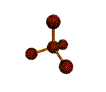##Mr. Rapp's Chemistry Help Site
 Grades  Graphing Tips  Online 3-D Laboratory  Reference Desk  AP Chemistry Test  News from Science  Links  After School Help  Fun Stuff  Teachers' Lounge    BHSS Chemistry  E-mail Mr. RappStudy Guide:  Chapter 21, Balancing Equations Students should be able to... Balance molecular equations know terms: reactants, products, solid, liquid, gas, aqueous, yields Know symbols used in equations. (solid, liquid, gas, aqueous, yields, etc.) Define: reactants, products Identify type of reaction (synthesis, decomposition, single replacement, double replacement, oxidation-reduction) How To Balance Chemical Equations 1. Reactants go on the left hand side and products go on the right hand side of a chemical equation. Be sure to write the correct formulas for the reactants and products. · Remember atoms are conserved in a chemical reaction. ie they are neither created or destroyed. So all the atoms in the reactants must end up somewhere among the products. 2. Count the number of atoms of each element, compound or ion in the reactants and products. If they are not equal proceed further. 3. Balance the atoms one at a time by placing coefficients in front of the formula so that the numbers of atoms of each element are equal on both sides of the equation. Remember atoms may exist in an element, compound or ion. · It is usually easier to start with the atoms that occur in only one substance on each side of the equation. · Balance the atoms that occur in compounds before attempting to balance atoms that occur in elemental form. e.g. H2, O2 or Cl2 · To make it easier if a polyatomic ion appears unchanged on both sides of the equation treat it as a whole unit. · When no coefficient is written in front of a formula it is assumed to be 1. 4. Numbers appearing in the formulas are known as subscripts. These can never be changed when balancing the equation or you will change the identity of the substance. · Remember with subscripts, any number to the right of parentheses multiplies each subscript within the parentheses. eg Fe2(SO4)3 contains 2 Fe atoms, 3 S atoms and 12 O atoms. 5. Finally make sure that all the coefficients are in the smallest possible whole number ratio. Balancing Chemical Equations (another tutorial) When writing chemical equations, students should already be familiar with writing chemical formulas. We use formulas to write chemical equations. A chemical equation is a shorthand method for describing a chemical change. The symbols and formulas are used to indicate the substances involved in the change. In order to balance an equation, we must know what the reactants and products are as well as the formulas of both. The formula of reactant(s) are found on the left hand side of the equation and the formula of the product(s) are found on the right hand side of the equation. The arrow is read as "yields" and the plus sign is read as "and". When a plus sign appears on the left side, it implies "reacts with". Ex. A + B --------> C + D reactants products Note that when balancing chemical reactions The Law of Conservation of Mass must be obeyed. This law states that during a chemical reaction matter is neither created nor destroyed. What this means is that there must be as many atoms of each type on the left-hand side as on the right-hand side when the equation is balanced. The proper coefficients are placed in front of the formulas in order to balance the numbers of atoms on each side of the equation. Students should follow the steps below in the order they are listed when balancing equations. · Step 1. Balance all atoms other than oxygen and hydrogen · Step 2. Balance all oxygen · Step 3. Balance hydrogen Remember that subscripts in a correct formula can not be changed in order to made an equation balance. Balance and classify these equations: 1. _____Ca(OH)2(s) + _____HCl(aq) ---> ____CaCl2(aq) + _____H2O(l) 2. _____FeCl3(aq) + _____(NH4)2S(aq) ---> _____Fe2S3(s) + _____NH4Cl(aq) 3. _____KNO3 (s) ---> _____KNO2 (s) + _____O2(g) 4. _____Ag2O(s) ---> _____Ag(s) + _____O2(g) 5. _____C4H10(g) + _____O2(g) ---> _____CO2(g) + _____H2O(g) 6. _____Br2(aq) + _____KI(aq) ---> _____I2(aq) + _____KBr(aq) 7. _____AsCl3(aq) + _____H2S (aq) ---> _____As2S3 (s) + _____HCl 8. _____C5H12O (l) + _____O2(g) --> ______CO2(g) + _____H2O (g) 9. _____Al(s) + _____H2SO4 (aq) ---> _____Al2(SO4)3(aq) + _____H2(g) 10. _____Fe(s) + _____Cl2(g) ---> _____FeCl3(s) 11. C5H12 + O2 -----> CO2 + H2O 12. NH3 + O2 -----> N2 + H2O 13. KOH + H2SO4 -----> K2SO4 + H2O 14. N2H4 + N2O4 -----> N2 + H2O 15. F2 + H2O -----> HF + O2 16. Na2O + H2O -----> NaOH 17. Na2CO3 + HNO3 -----> H2O + CO2 + NaNO3 18. Ca3P2 + H2O -----> PH3 + Ca(OH)2 19. IBr + NH3 -----> NI3 + NH4Br 20. NH4NO3 -----> N2 + H2O + O2E-mail Mr. Rapp | BHSS Chemistry Web SiteLast updated on October 14, 2005 9:09 AM